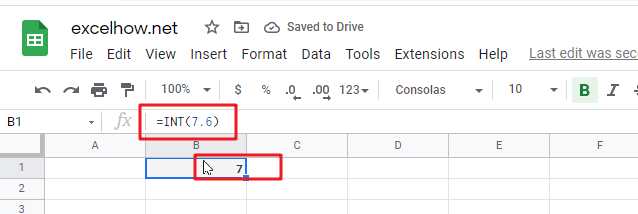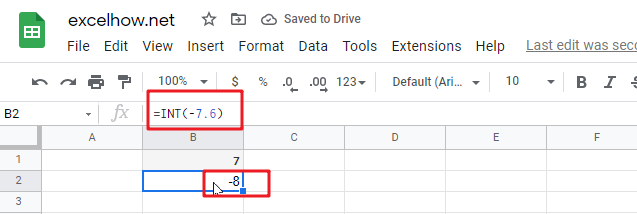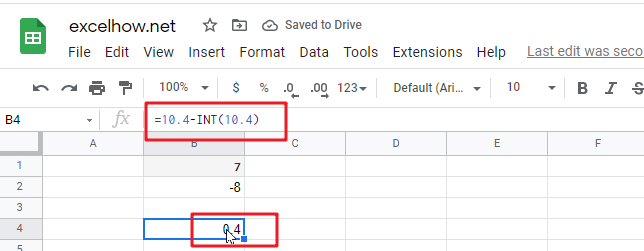This post will guide you how to use Google Sheets INT function with syntax and examples.

## Description

The Google Sheets INT function returns the integer portion of a given number. And it will round a given number down to the nearest integer.  And the INT function rounds down, so if you provide a negative number, the returned value will become more negative. It means that it will return the first negative number that is less than or equal to the original number. Such as, INT(-5.9), it will return -6.

The purpose of this function is to get the integer part of a number in google sheets. And its returned value is the integer part of the number.

The INT function is a build-in function in Google Sheets and it is categorized as a MATH function.

## Syntax

The syntax of the INT function is as below:

= INT (number)

Where the INT function argument is:

• numberThis is a required argument. The real number that you want to round down to an integer.

## Google Sheets INT Function Examples

The below examples will show you how to use google sheets INT Function to truncate a given number down to the nearest integer.

1# get the integer portion of a given number 7.6, enter the following formula in Cell B1.

`=INT(7.6)`2# rounding a given negative -7.6 down to the nearest integer, enter the following formula in Cell B2.

`=INT(-7.6)`3# get the decimal part of a positive number 10.4, enter the following formula in Cell B3.

`=10.4-INT(10.4)`Related Posts

This post will guide you how to use Google Sheets FLOOR function with syntax and examples. Description The Google Sheets FLOOR function returns a number rounded down to the nearest multiple of significance. So it will return a rounded number. ...

This post will guide you how to use Google Sheets EXP function with syntax and examples. Description The Google Sheets EXP function returns e raised to the power of a number.  The constant e is equal to 2.71828182845904, and it ...

This post will guide you how to use Google Sheets FACT function with syntax and examples. Description The Google Sheets FACT function returns the factorial of a given number.  The factorial of a given number is equal to 1*2*3…* number. ...

This post will guide you how to use Google Sheets EVEN function with syntax and examples. Description The Google Sheets EVEN function rounds a given number up to the nearest even integer. You can use the EVEN function to return ...

This post will guide you how to use Google Sheets COUNTBLANK function with syntax and examples. Description The Google Sheets COUNTBLANK function use to count the number of empty cells in a range of cells. You can use the COUNTBLANK ...

This post will guide you how to use Google Sheets DEGREES function with syntax and examples. Description The Google Sheets DEGREES function converts radians into degrees. And it will return a numeric value. For examples, if you pass the PI() function into ...

This post will guide you how to use Google Sheets COSH function with syntax and examples. Description The Google Sheets COSH function returns the hyperbolic cosine of a given angle. The purpose of this function is used to get hyperbolic ...